# Design and implement synchronous dual port 128×8 RAM using VHDL. Dual port RAM supports simultane...

Design and implement synchronous dual port 128×8 RAM using VHDL. Dual port RAM supports simultaneous read and write operations.

library ieee;
use ieee.std_logic_1164.all;
use ieee.std_logic_unsigned.all;

entity sync_dual_port_ram is
port (   clock    : in std_logic;
port0_en: in std_logic;
port1_en: in std_logic;
rd_wr   : in std_logic;
din0   : in std_logic_vector(7 downto 0);
din1   : in std_logic_vector(7 downto 0);
addr0   : in std_logic_vector(6 downto 0);
addr1   : in std_logic_vector(6 downto 0);
dout0   : out std_logic_vector(7 downto 0);
dout1   : out std_logic_vector(7 downto 0)
);
end sync_dual_port_ram;

architecture arch of sync_dual_port_ram is

type memory is array (0 to 127) of std_logic_vector(7 downto 0);
signal ram : memory;

begin

process(clock)
begin
if rising_edge(clock) then
if (rd_wr = '0') then
if (port0_en = '1') then
end if;
if (port1_en = '1') then
end if;
else
end if;
end if;

end process;

process(clock)
begin
if rising_edge(clock) then
end if;
end process;

end arch;

----------------------------------------------------------------------------------------------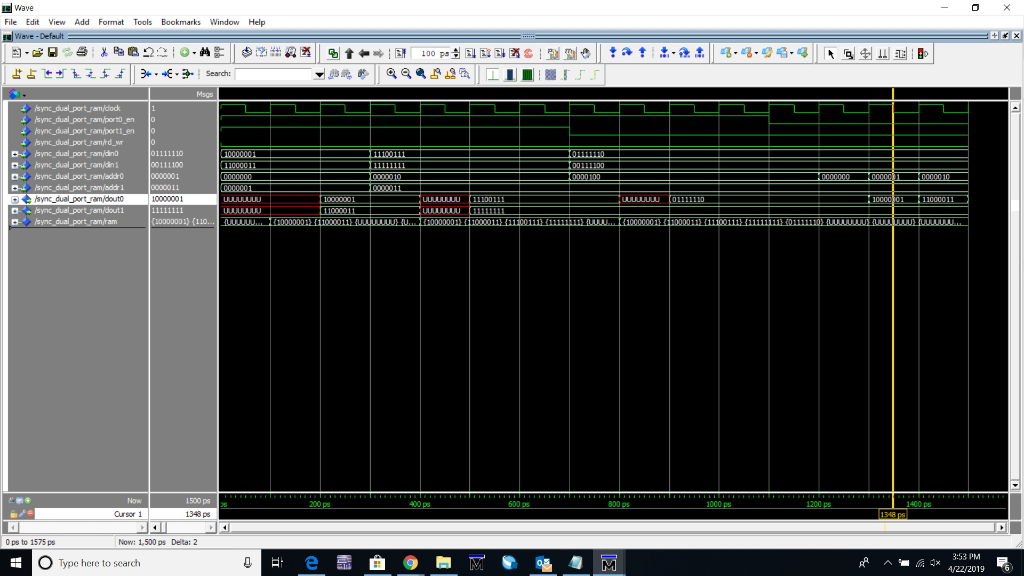##### Add Answer of: Design and implement synchronous dual port 128×8 RAM using VHDL. Dual port RAM supports simultane...
Similar Homework Help Questions
• ### Part Ii Using positive logic, and an input variable x, design a MOD 4 Synchronous Counter to coun...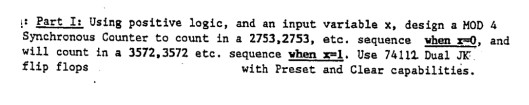Part Ii Using positive logic, and an input variable x, design a MOD 4 Synchronous Counter to count in a 2753,2753, etc. sequence vhen -0, and will count in a 3572.3572 etc. sequence vhen 1. Use 74112 Dual JK flip flops with Preset and Clear capabilities Part Ii Using positive logic, and an input variable x, design a MOD 4 Synchronous Counter to count in a 2753,2753, etc. sequence vhen -0, and will count in a 3572.3572 etc. sequence vhen...

• ### Design and implement a 8 or 6 Port Dip Switch that control 4 LED Lights Components: Dip Switch, L...

Design and implement a 8 or 6 Port Dip Switch that control 4 LED Lights Components: Dip Switch, LEDs, Resistors Description: Using a dipswitch create an application that uses bitwise operator to shift the bits to the left one bit at a time. Turning on one LED at a time that corresponds to the dipswitch position. After the bits are shifted 4 positions, start shifting the bits to the right turning the light on and off in the reverse order...

• ### A Y = A+ B 1 1 1 1 1 1 Apply the idea of the truth table above and design a 3-bit comparator using XOR or XNOR gates of...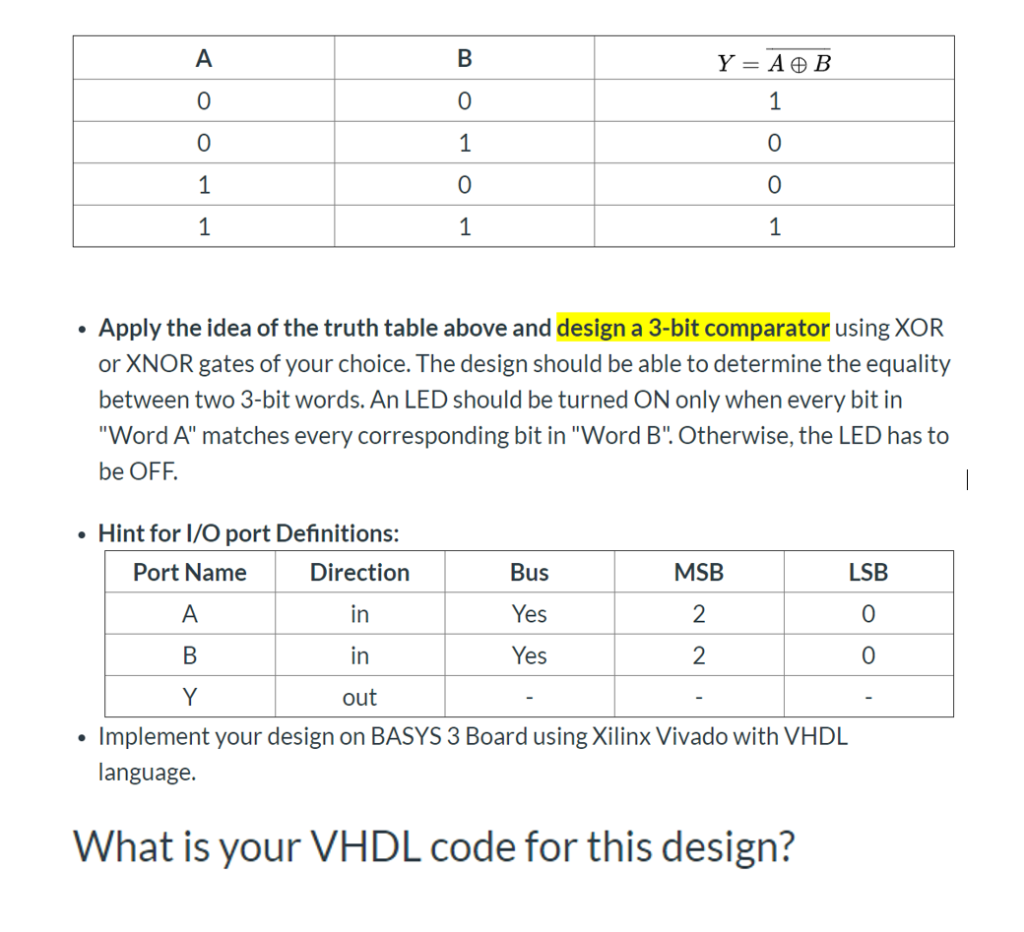A Y = A+ B 1 1 1 1 1 1 Apply the idea of the truth table above and design a 3-bit comparator using XOR or XNOR gates of your choice. The design should be able to determine the equality between two 3-bit words. An LED should be turned ON only when every bit in "Word A" matches every corresponding bit in "Word B". Otherwise, the LED has to be OFF Hint for I/O port Definitions: Port Name MSB...

• ### Exercise (2): Implement the digital circuit in Fig. 2 using VHDL. D Q D, 2 9 CLKJ Figure 2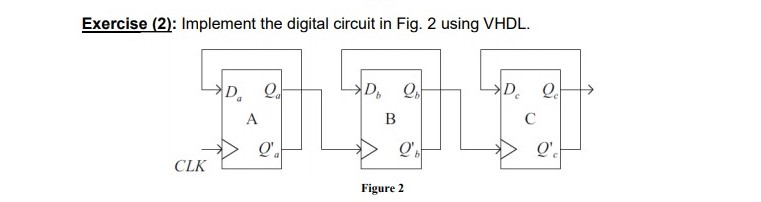please explain that, compile it and give me a solution as text Exercise (2): Implement the digital circuit in Fig. 2 using VHDL. D Q D, 2 9 CLKJ Figure 2 Exercise (2): Implement the digital circuit in Fig. 2 using VHDL. D Q D, 2 9 CLKJ Figure 2

• ### Design a dual 8-to-1 line multiplexer using a 3-to-8 line decoder and two 8X2 AND-ORS.

Design a dual 8-to-1 line multiplexer using a 3-to-8 line decoder and two 8X2 AND-ORS.

• ### Write a VHDL module that implements a combinational dircuit that indicates the number of days in a given month. Notes: Q4) .. The month input is a single port (4-bit number The Leap Year port indicat...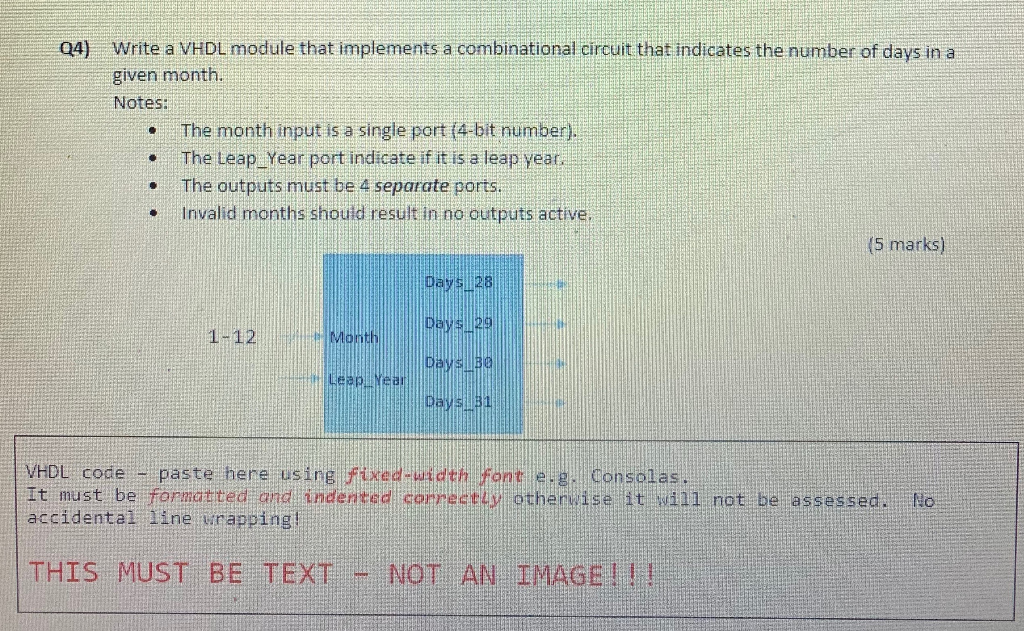Write a VHDL module that implements a combinational dircuit that indicates the number of days in a given month. Notes: Q4) .. The month input is a single port (4-bit number The Leap Year port indicate if it is a leap yeat . The outputs must be 4 separate ports .Invalid months shouid result in no outputs active. (5 marks) di 1緋 VHDL code paste here using fixed-uidth gth Fonr e consolas Et must be formanted and indented correstty otherwise...

• ### (20 pts)VHDL. Implement the logic circuit specified in the following truth table by using a 4:1 m...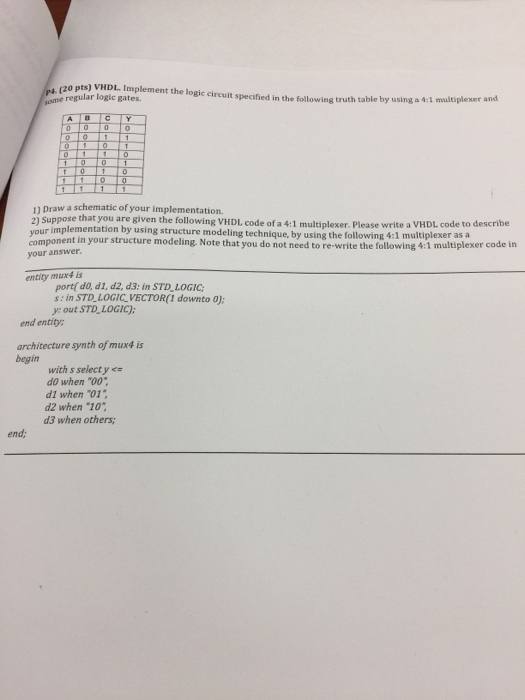(20 pts)VHDL. Implement the logic circuit specified in the following truth table by using a 4:1 mulitiplexer ome regular logic gates. 11 Draw a schematic of your implementation. 2) Suppose that you are given the following VHDL code of a 4:1 multiplexer. Please write a VHDL code to describe your implementation by using structure modeling technique, by using the following 4:1 multiplexer asia your answer component in your structure modeling. Note that you do not need to re-write the following...

• ### Experiment #3 Design and create simulation waveform for an 8-bit up/down synchronous (rising edge of clock) binary coun...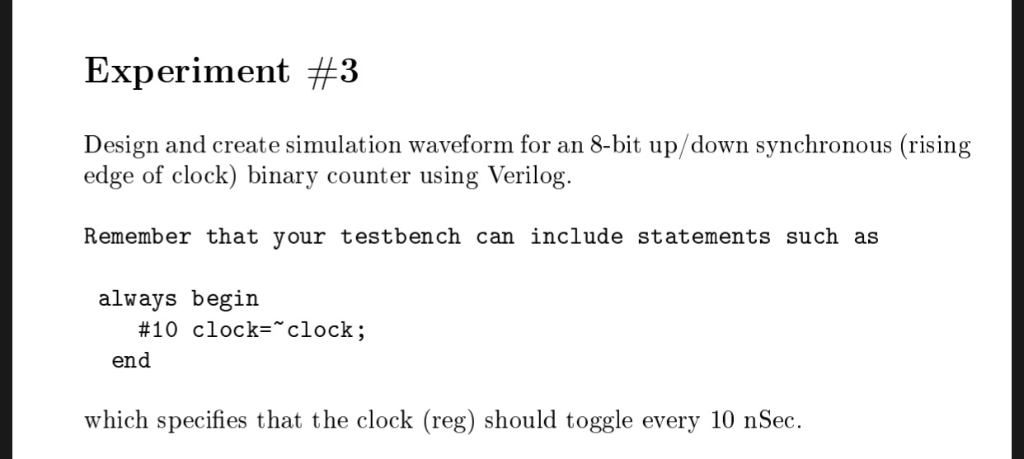Experiment #3 Design and create simulation waveform for an 8-bit up/down synchronous (rising edge of clock) binary counter using Verilog Remember that your testbench can include statements such as always begin #10 clock="clock; end which specifies that the clock (reg) should toggle every 10 nSec Experiment #3 Design and create simulation waveform for an 8-bit up/down synchronous (rising edge of clock) binary counter using Verilog Remember that your testbench can include statements such as always begin #10 clock="clock; end which...

• ### i. Design and test a 3-to-8 decoder with active-low outputs using VHDL/HDL.    Demonstrate your outputs in the BASYS board. (Note: Capture the pictures of your    output and add in in your answer scr...

i. Design and test a 3-to-8 decoder with active-low outputs using VHDL/HDL.    Demonstrate your outputs in the BASYS board. (Note: Capture the pictures of your    output and add in in your answer script)       ii. Include (screenshot) VHDL codes and .xdc file modification in your answer script.      iii. Develop a truth table following your outputs.

• ### using J-K flip-flops, design a synchronous counter to produce the following repeating sequence 0,6,2,4,0 and prove it in Multisim.

using J-K flip-flops, design a synchronous counter to produce the following repeating sequence 0,6,2,4,0 and prove it in Multisim.

Need Online Homework Help?